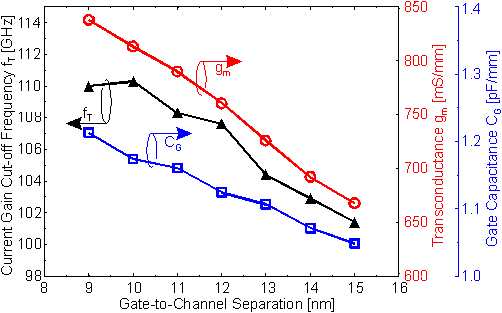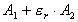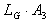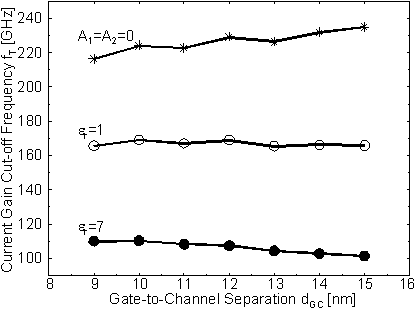Next: 6.3.2.4 Dependence of fT and fmax on LR Up: 6.3.2 RF Performance Previous: 6.3.2.2 Contributions to the Gate Capacitance

6.3.2.3 Dependence of fT on dGC

It was shown in  Figure 6.44 that fT can be increased for small LG by a reduction of dGC. This is shown in more detail in  Figure 6.46 for the case LG = 120 nm. Although gm is increased from 668 mS/mm for dGC = 15 nm to 838m S/mm for dGC = 9 nm only a moderate improvement of fT from 101 GHz to 110 GHz is obtained. This is due to the increase of CG from 1.05 pF/mm to 1.21 pF/mm as depicted in  Figure 6.46.Figure 6.46 Dependence of gm, CG, and fT on dGC. The strong increase of CG with a reduction of dGC almost compensates the improvements in fT. Only a moderate increase is obtained.

All three characteristics are expected to decrease monotonously thus, the local maximum in fT is believed to be result of some discretization error which is reflected in the CG(dGC) characteristics.

According to (62) CG is the sum of three different contributions. The comparison between devices with different dGC revealed that only A2 depends strongly on dGC. Thus the relative improvement of fT versus dGC is dependent on the ratio between the constant part of CG, i. e., and the part dependent on dGC, i. e.. This is illustrated in  Figure 6.47.Figure 6.47 fT versus dGC for different assumptions on the gate capacitance. For er = 7 the characteristics of  Figure6.46 is obtained. er = 1 represents unpassivated devices. The slope of the characteristics is even positive if only the contribution of CG dependent on LG is considered.

fT versus dGC for a passivated device is shown in  Figure 6.47. In addition the fT for an unpassivated device is given. In this case fT is almost independent of dGC in the given range from dGC = 9 nm to dGC = 15 nm. A theoretical fT can be calculated by setting the constant contributions of CG to zero, i. e. A1 = A2 = 0 such that fT reduces to fT = gm/2pLGA3. This way the impact of dGC on gm and CG can be isolated. The slope of the corresponding characteristics is positive. In other words, A3 increases more rapidly with a reduction of dGC than gm.

This in turn explains the crossed fT(LG) characteristics for the two different distances dGC. For large LG the increase of CG overcompensates the improvements in gm. For smaller LG the quotient of the constant part of CGoveris increased such that the improvement in gm is not fully compensated.Next: 6.3.2.4 Dependence of fT and fmax on LR Up: 6.3.2 RF Performance Previous: 6.3.2.2 Contributions to the Gate Capacitance

Helmut Brech
1998-03-11